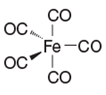# Problem: For the following complex, determine the oxidation state of the metal, the total number of valence electrons it possesses, and state whether the metal is coordinatively saturated or unsaturated.a) Oxidation State = +5 , Valence Electrons = 18 , Unsaturatedb) Oxidation State = 0 , Valence Electrons = 18 , Saturatedc) Oxidation State = +3 , Valence Electrons = 18 , Saturatedd) Oxidation State = +2 , Valence Electrons = 18 , Saturated

###### FREE Expert Solution
85% (379 ratings)
###### Problem Details

For the following complex, determine the oxidation state of the metal, the total number of valence electrons it possesses, and state whether the metal is coordinatively saturated or unsaturated.a) Oxidation State = +5 , Valence Electrons = 18 , Unsaturated

b) Oxidation State = 0 , Valence Electrons = 18 , Saturated

c) Oxidation State = +3 , Valence Electrons = 18 , Saturated

d) Oxidation State = +2 , Valence Electrons = 18 , Saturated

Frequently Asked Questions

What scientific concept do you need to know in order to solve this problem?

Our tutors have indicated that to solve this problem you will need to apply the Coordination Complexes concept. You can view video lessons to learn Coordination Complexes. Or if you need more Coordination Complexes practice, you can also practice Coordination Complexes practice problems.

What is the difficulty of this problem?

Our tutors rated the difficulty ofFor the following complex, determine the oxidation state of ...as medium difficulty.

How long does this problem take to solve?

Our expert Organic tutor, Jules took 1 minute and 32 seconds to solve this problem. You can follow their steps in the video explanation above.